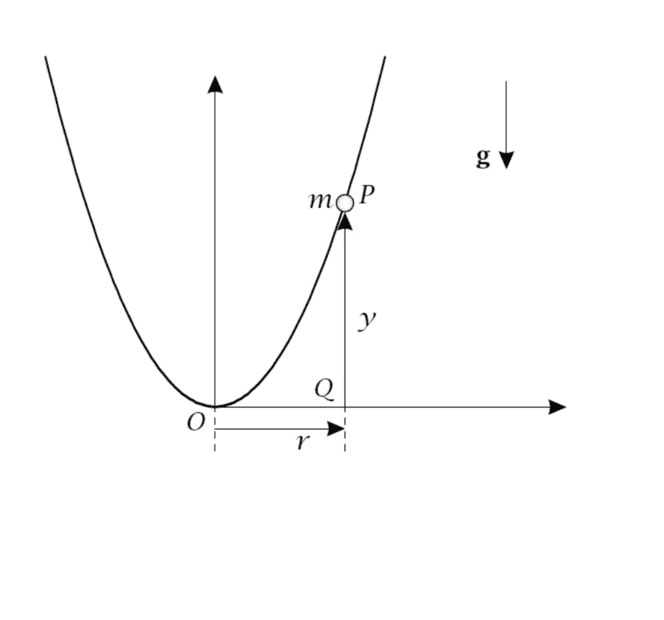# Finding the differential equation of motion

• dbeckam
The differential equation of motion is y" + a*y' + b*y = F(t).The energy is conserved and equal to its initial value, ##E(r,\dot{r}) = E_0##.

#### dbeckam

Thread moved from the technical forums to the schoolwork forums
Summary:: Differential equation of motion, parabola

Hi. I've tried resolve this problem but I have two doubts. The first is about the differential equation of motion because I can't simplify it to the form y" + a*y' + b*y = F(t). I'm not sure if what I got is right. My second doubt is that I cannot solve part b. Thank you in advance for your help.

In part a I got this: r"(R^2 + r^2) + (r'^2)r + g*r*R = 0.

Problem:
A particle of mass m slides without friction along a fixed track in the form of a parabola. The equation of the parabola is y = (r^2)/(2R). R is a constant, r is the distance between O and Q, y is the vertical distance. Initial conditions: r(t=0) = r_0 and r'(t=0) = 0.

a) Find the differential equation of motion in terms of the variable r.
b) Find an expression for (r')^2 in function of r.I agree with your equation of motion. The easiest way to find ##\dot{r}## is by considering the energy, which you should write as a function of ##r## and ##\dot{r}##. The energy is conserved and equal to its initial value, ##E(r,\dot{r}) = E_0##, which you can find from the initial conditions.

•dbeckam
dbeckam said:
I can't simplify it to the form y" + a*y' + b*y = F(t)
Why do you think it should be possible to simplify it to this form? This form is in essence a driven harmonic oscillator with a friction term.

•dbeckam
Orodruin said:
Why do you think it should be possible to simplify it to this form? This form is in essence a driven harmonic oscillator with a friction term.
Yes, I understand. Thanks!

ergospherical said:
I agree with your equation of motion. The easiest way to find ##\dot{r}## is by considering the energy, which you should write as a function of ##r## and ##\dot{r}##. The energy is conserved and equal to its initial value, ##E(r,\dot{r}) = E_0##, which you can find from the initial conditions.
I already resolved it. Thank you very much.